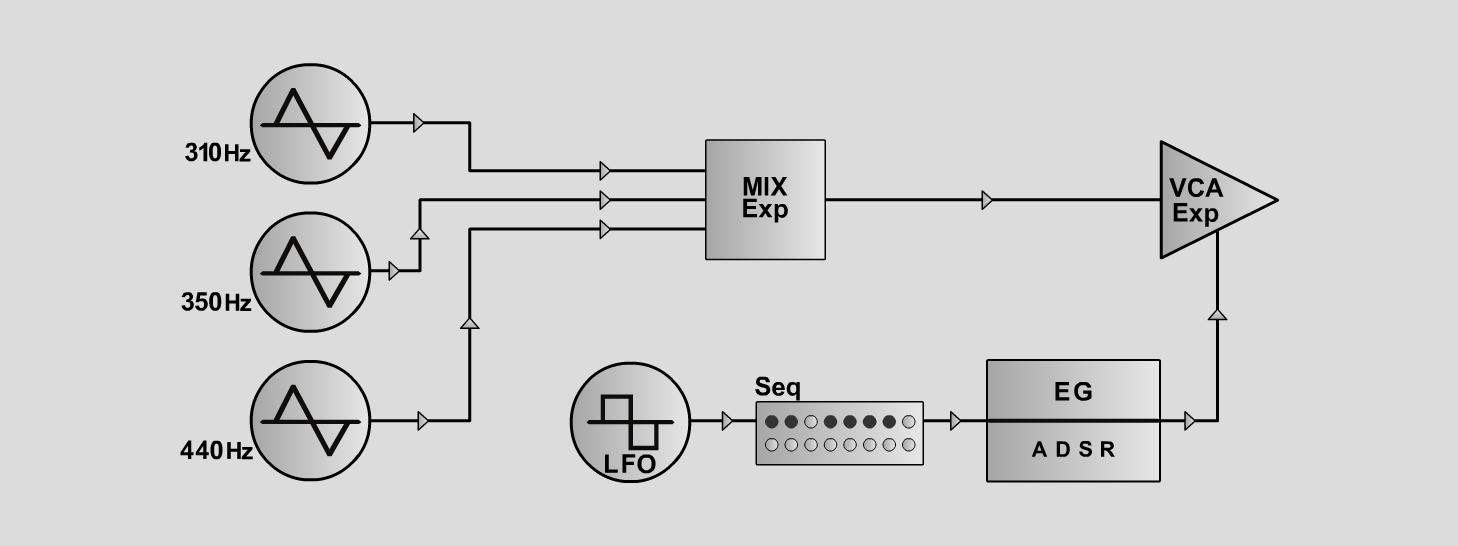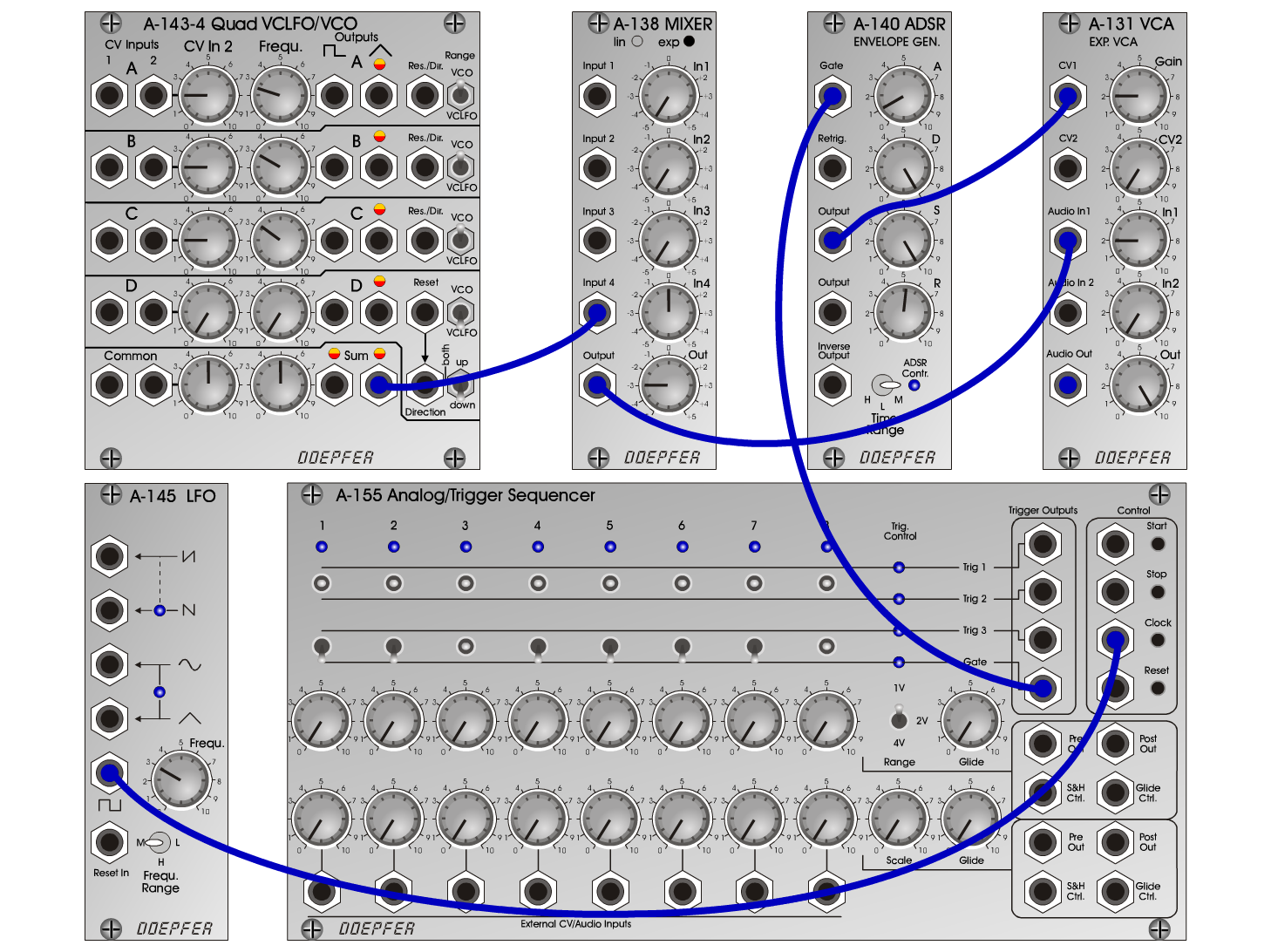Train HornFXD o e p f e r A - 100 Connections: Settings: Train Horn 1: A-143-4 (Sum Triangle) <=> A-138exp (Input 4)  A-138exp (Output) <=> A-131 (Audio In)  A-145 (Square) <=> A-155 (Clock)  A-155 (Gate) <=> A-140 (Gate)  A-140 (Output) <=> A-131 (CV1) A-143-4 (A: CV In 2 = 2, Frequ = 2.6, Range = VCO,    B: CV In 2 = 2, Frequ = 3, Range = VCO,    C: CV In 2 = 2, Frequ = 3.2, Range = VCO,    D: CV In 2 = 0, Frequ = 0, Range = VCLFO, Direction = down,    Common: CV In 2 = 5, Frequ = 5)  A-138exp (In 4 = 5, Out = 2)  A-145 (Frq = 3, Range = M)  A-155 (Gate: 1 = down, 2 = down, 3 = mid, 4 = down,    5 = down, 6 = down, 7 = down, 8 = mid)  A-140 (A = 1, D = 10, S = 10, R = 5.2, Range = M)  A-131 (Gain = 2, Audio In 1 = 2, Audio Out = 10) Train Horn 2: A-143-4 Frequencies:  A = 310 Hz  B = 370 Hz  C = 495 Hz Train Horn 3: A-143-4 Frequencies:  A = 310 Hz  B = 370 Hz  C = 465 Hz Train Horn 4: A-143-4 Frequencies:  A = 280 Hz  B = 330 Hz  C = 440 Hz Train Horn 5: A-143-4 Frequencies:  A = 295 Hz  B = 395 Hz  C = 440 Hz Train Horn 6: A-143-4 Frequencies:  A = 255 Hz  B = 310 Hz  C = 440 Hz Josef MuellerSound samples Train Horn 1 Train Horn 2 Train Horn 3 Train Horn 4 Train Horn 5 Train Horn 6